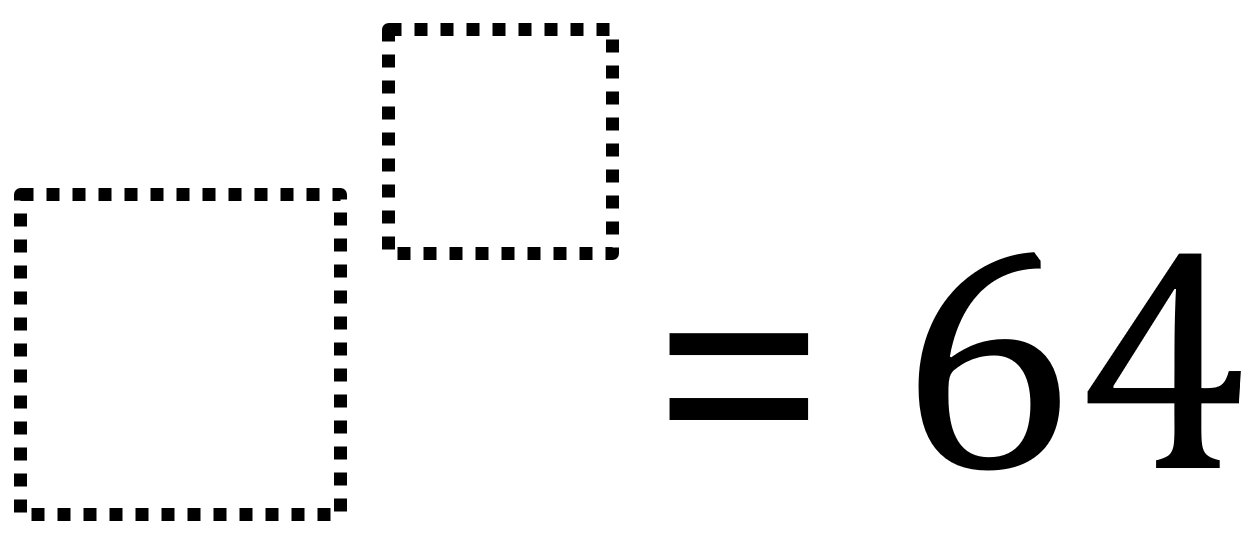# Exponent Exploration

Directions: Using the digits 1 to 9, at most one time each, fill in the boxes to make two true number sentences.### Hint

What are the factors of 64?
What are the prime factors of 64?
What numbers times itself multiple times can equal 64?

2^6
4^3
8^2

Source: Robert Kaplinsky

## Square Root Expression 2

Directions: Using the digits 0 to 9 at most one time each, place a digit …

1.•2.